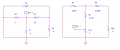# Time Constant

Joined Feb 16, 2010
112
I need help finding the time constant for the circuit attached. I've written the nodal equations at the two significant nodes and have come up with an answer by substituting the non-cap node into the other to come up with a differential equation. I am just seeking clarification.

#### Ghar

Joined Mar 8, 2010
655
Personally I'd find it by doing a delta-wye transformation or by using the extra element theorem... either way the answer is 3.33s

http://en.wikipedia.org/wiki/Delta_wye

You'd transform R1, R2 and R3 into a Y connection...Now you get:
$Rth = R5 + R7 || (R4 + R6) = 0.667 \Omega$

Then the time constant is of course just RC = 3.33 s

Plot showing that's correct and they're equivalent:Or you can use the extra element theorem, by removing R1:

$Rth = (R with R1 open)\frac{1 + \frac{R seen by R1 with C1 short}{R1}}{1 + \frac{R seen by R1 with C1 open}{R1}}\\ Rth = R2 || (R3 + R4) \frac{1 + \frac{R3 || R4}{R1}}{1 + \frac{(R2 + R3)||R4}{R1}} Rth = 2||4.2 \frac{1 + 4||0.2}{1 + 2.2||4} = 0.667 \Omega$

$Rth = R2 || (R1 || R4 + R3) = 2 || (1||4 + 0.2) = 2 || 1 = 0.667 \Omega$